# 【算法面试】leetcode最常见的150道前端面试题 --- 简单题下（44题）

## 二叉树前中后遍历套路详解

root节点是A节点（下图的A节点），然后让你按照下图数字的顺序依次打印出节点。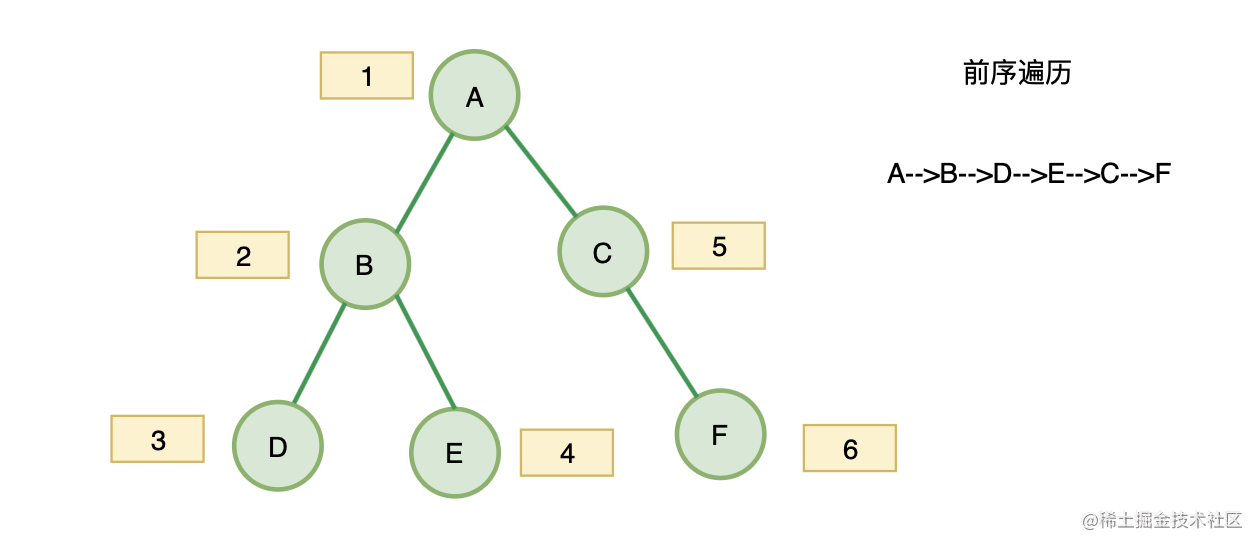``````var Traversal = function(root) {
const stack = [];
while (root || stack.length){
while(root){
stack.push(root);
root = root.left;
}
root = stack.pop();
root = root.right;
}
return res;
};

• A、B、D入栈，
• D出栈
• B出栈
• E入栈
• E出栈
• A出栈
• C入栈
• C出栈
• F入栈
• F出栈

``````var preorderTraversal = function(root) {
// 初始化数据
const res =[];
const stack = [];
while (root || stack.length){
while(root){
res.push(root.val);
stack.push(root);
root = root.left;
}
root = stack.pop();
root = root.right;
}
return res;
};

``````var preorderTraversal = function(root) {
// 初始化数据
const res =[];
const stack = [];
while (root || stack.length){
while(root){
stack.push(root);
root = root.left;
}
root = stack.pop();
res.push(root.val);
root = root.right;
}
return res;
};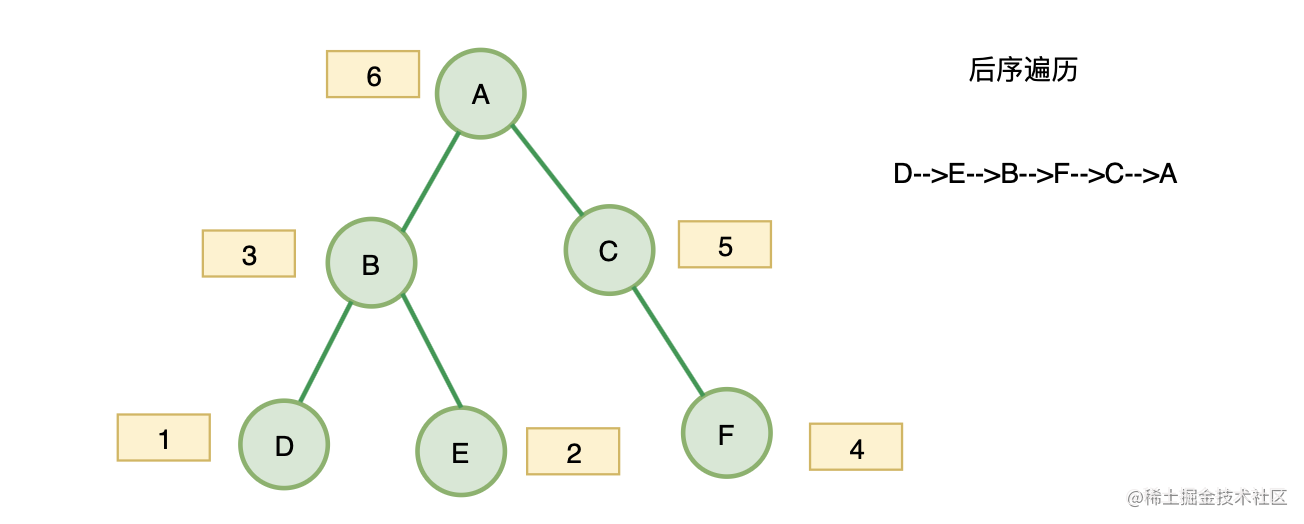``````var postorderTraversal = function(root) {
// 初始化数据
const res =[];
const stack = [];
while (root || stack.length){
while(root){
stack.push(root);
res.unshift(root.val);
root = root.right;
}
root = stack.pop();
root = root.left;
}
return res;
};

## 对称二叉树

``````    1
/ \
2   2
/ \ / \
3  4 4  3

``````    1
/ \
2   2
\   \
3    3

• 判断两个指针当前节点值是否相等
• 判断 `A` 的右子树与 `B` 的左子树是否对称
• 判断 `A` 的左子树与 `B` 的右子树是否对称
``````function isSame(leftNode, rightNode){
if(leftNode === null && rightNode === null) return true;
if(leftNode === null || rightNode === null) return false;
return leftNode.val === rightNode.val && isSame(leftNode.left, rightNode.right) && isSame(leftNode.right, rightNode.left)
}
var isSymmetric = function(root) {
if(!root) return root;
return isSame(root.left, root.right);
};

## 二叉树的最大深度

• 只要遍历到这个节点既没有左子树，又没有右子树的时候
• 说明就到底部了，这个时候如果之前记录了深度，就可以比较是否比之前记录的深度大，大就更新深度
• 然后以此类推，一直比较到深度最大的
``````var maxDepth = function(root) {
if(!root) return root;
let ret = 1;
function dfs(root, depth){
if(!root.left && !root.right) ret = Math.max(ret, depth);
if(root.left) dfs(root.left, depth+1);
if(root.right) dfs(root.right, depth+1);
}
dfs(root, ret);
return ret
};

## 将有序数组转化为二叉搜索树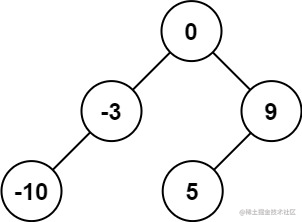``````输入：nums = [-10,-3,0,5,9]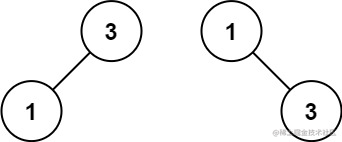``````输入：nums = [1,3]

1 <= nums.length <= 104
-104 <= nums[i] <= 104
nums 按 严格递增 顺序排列

• 构建一颗树包括：构建`root、构建 root.left 和 root.right`
• 题目要求"高度平衡" — 构建 `root` 时候，选择数组的中间元素作为 `root `节点值，即可保持平衡。
• 递归函数可以传递数组，也可以传递指针，选择传递指针的时候： l r 分别代表参与构建BST的数组的首尾索引。
``````var sortedArrayToBST = function(nums) {
};
const toBST = function(nums, l, r){
if( l > r){
return null;
}
const mid = l + r >> 1;
const root = new TreeNode(nums[mid]);
root.left = toBST(nums, l, mid - 1);
root.right = toBST(nums, mid + 1, r);

return root;
}

## 20. 有效的括号

``````示例 1：

1. 右括号前面，必须是相对应的左括号，才能抵消！
2. 右括号前面，不是对应的左括号，那么该字符串，一定不是有效的括号！

``````var isValid = function(s) {
const map = { '{': '}', '(': ')', '[': ']' };
const stack = [];
for(let i of s){
if(map[i]){
stack.push(i);
} else {
if(map[stack[stack.length - 1]] === i){
stack.pop()
}else{
return false;
}
}
}
return stack.length === 0;
};

## 155、 最小栈

• push(x) —— 将元素 x 推入栈中。
• pop() —— 删除栈顶的元素。
• top() —— 获取栈顶元素。
• getMin() —— 检索栈中的最小元素。

``````示例:

MinStack minStack = new MinStack();
minStack.push(-2);
minStack.push(0);
minStack.push(-3);
minStack.getMin();   --> 返回 -3.
minStack.pop();
minStack.top();      --> 返回 0.
minStack.getMin();   --> 返回 -2.

pop、top 和 getMin 操作总是在 非空栈 上调用。

``````var MinStack = function() {
this.stack = [];
};

MinStack.prototype.push = function(x) {
this.stack.push(x);
};

MinStack.prototype.pop = function() {
this.stack.pop();
};

MinStack.prototype.top = function() {
return this.stack[this.stack.length - 1];
};

• 开始我们向stack push -2
• 此时辅助栈minStack，因为此时stack最小的是-2，也push -2
• stack push 0
• 此时辅助站minStack 会用 0 跟 -2对比，-2更小，minstack会push -2
• stack push -3
• 此时辅助站minStack 会用 -3 跟 -2对比，-3更小，minstack会push -3

``````var MinStack = function() {
this.stack = [];
// 辅助栈
this.minStack = [];
};

MinStack.prototype.push = function(x) {
this.stack.push(x);
// 如果是第一次或者当前x比最小栈里的最小值还小才push x
if(this.minStack.length === 0 || x < this.minStack[this.minStack.length - 1]){
this.minStack.push(x)
} else {
this.minStack.push( this.minStack[this.minStack.length - 1])
}
};

MinStack.prototype.pop = function() {
this.stack.pop();
this.minStack.pop();
};

MinStack.prototype.top = function() {
return this.stack[this.stack.length - 1];
};

MinStack.prototype.getMin = function() {
return this.minStack[this.stack.length - 1];
};

## 53. 最大子序和

``````示例 1：

• 这道题可以用动态规划来解决，关键是找动态转移方程
• 我们动态转移方程中，dp表示每一个nums下标的最大自序和，所以dp[i]的意思为：包括下标i之前的最大连续子序列和为dp[i]。

dp[i]只有两个方向可以推出来：

• 1、如果dp[i - 1] < 0，也就是当前遍历到nums的i，之前的最大子序和是负数，那么我们就没必要继续加它了，因为dp[i] = dp[i - 1] + nums[i] 会比nums[i]更小，所以此时还不如dp[i] = nums[i]，就是目前遍历到i的最大子序和呢
• 2、同理dp[i - 1] > 0，说明nums[i]值得去加dp[i - 1]，此时回避nums[i]更大

``````var maxSubArray = function(nums) {
let res = nums;
const dp = [nums];
for(let i=1;i < nums.length;i++){
if(dp[i-1]>0){
dp[i]=nums[i]+dp[i-1]
}else{
dp[i]=nums[i]
}

res=Math.max(dp[i],res)
}
return res
};

## 70. 爬楼梯

``````示例 1：

1.  1 阶 + 1 阶
2.  2 阶

1.  1 阶 + 1 阶 + 1 阶
2.  1 阶 + 2 阶
3.  2 阶 + 1 阶

``````var climbStairs = function(n) {
const dp = {};
dp = 1;
dp = 2;
for(let i = 3; i <= n; i++){
dp[i] = dp[i-1] + dp[i-2]
}
return dp[n]
};

## 66. 加一

``````示例 1：

• 需要有一个进位的变量carry记录到底进位是几
• 还需要一个每次迭代都重置和的变量sum来帮我们算是否进位，以及进位后的数字

``````var plusOne = function(digits) {
let carry = 1; // 进位（因为我们确定+1，初始化进位就是1）
for(let i = digits.length - 1; i >= 0; i--){
let sum = 0; // 这个变量是用来每次循环计算进位和digits[i]的值的
sum = digits[i] + carry;
digits[i] = sum % 10; // 模运算取个位数
carry = (sum / 10) | 0; //  除以10是取百位数，并且｜0表示舍弃小数位
}
if(digits === 0) digits.unshift(carry);
return digits
};

## 69 x的平方根

``````输入: 4

``````输入: 8

由于返回类型是整数，小数部分将被舍去。

``````const arr = [1, 2, 3, 4, 5, 6];
function getIndex1(arr, key) {
let low = 0;
const high = arr.length - 1;
while (low <= high) {
const mid = Math.floor((low + high) / 2);
if (key === arr[mid]) {
return mid;
}
if (key > arr[mid]) {
low = mid + 1;
} else {
height = mid - 1;
}
}
return -1;
}
console.log(getIndex1(arr, 5)); // 4

``````var mySqrt = function(x) {
let [l , r] = [0, x];
let ans = -1;
while(l <= r) {
const mid = (l + r) >> 1;
if(mid * mid > x){
r = mid - 1
} else if(mid * mid < x){
ans = mid; // 防止越界
l = mid + 1;
} else {
ans = mid;
return ans;
}
}
return ans;
};
};

## 171. Excel表序列号

``````A -> 1
B -> 2
C -> 3
...
Z -> 26
AA -> 27
AB -> 28
...

``````示例 1：

• 初始化结果 `ans = 0`，遍历时将每个字母与 `A` 做减法，因为 `A` 表示 `1`，所以减法后需要每个数加 `1`，计算其代表的数值 `num = 字母 - ‘A’ + 1`
• 因为有 26 个字母，所以相当于 `26 进制`，每 `26` 个数则向前进一位
• 所以每遍历一位则`ans = ans * 26 + num`
• 以 ZY 为例，Z 的值为 `26`，Y 的值为 `25`，则结果为 `26 * 26 + 25=701`
``````var titleToNumber = function(columnTitle) {
let ans = 0;
for(let i = 0; i < columnTitle.length; i++){
ans = ans * 26 + (columnTitle[i].charCodeAt() - 'A'.charCodeAt() + 1)
}
return ans;
};

## 172. 阶乘中的零

``````示例 1:

• 也就是 `5 * 4 * 3 * 2 * 1 = 120`，我们发现只有1个0，怎么产生的呢，主要造成者就是 2 * 5 构造了一个0

• 再看看10!

10! = 10 * 9 * 8 * 7 * 6 * 5 * 4 * 3 * 2 * 1 其中，除了10 = 2 * 5和本身有一对2 * 5，所以有两个0，这样这道题的规律就出来了，我们再精进一步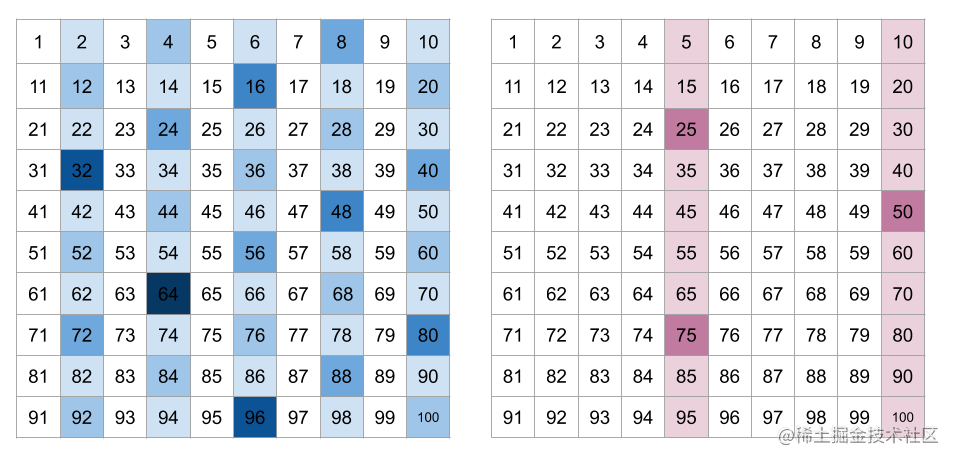``````var trailingZeroes = function (n) {
let r = 0;
while (n > 1) {
n = Math.floor(n / 5);
r += n;
}
return r;
};

## 190.颠倒二进制位

``````输入: 00000010100101000001111010011100

因此返回 964176192，其二进制表示形式为 00111001011110000010100101000000。

``````输入：11111111111111111111111111111101

因此返回 3221225471 其二进制表示形式为 10111111111111111111111111111111 。

1. 与预算 `&`
``````1 & 1 // 1的2进制最后一位是1，得到1
2 & 0 // 2的2进制最后一位是0，得到0
3 & 1 // 3的2进制最后一位是1，得到1
4 & 0 // 4的2进制最后一位是0，得到0

1. JavaScript 使用 32 位按位运算数(意思是我们的按位运算都会转成32位，你的数字不能超过32位，会出问题)
• JavaScript 将数字存储为 64 位浮点数，但所有按位运算都以 32 位二进制数执行。

• 在执行位运算之前，JavaScript 将数字转换为 32 位有符号整数。

• 执行按位操作后，结果将转换回 64 位 JavaScript 数。

1. `'<< 1' 运算`

• 2的2进制是 10，2 << 1 得到4， 4的2进制是100，所以比10多了个0
• 3的2进制是 11，3 << 1 得到6。 6的2进制是110，所以比11多了个0

``````var reverseBits = function (n) {
let result = 0
for (let i = 0; i < 32; i++) {
result = (result << 1) + (n & 1)
n = n >> 1
}
// 为什么要 >>> 0 呢，一位javascript没有无符号整数，全是有符号的
// 不>>>0的话，得出来的值是负数，但是无符号整数是没有符号的
// javascript 有符号转化为无符号的方法就是>>>0
return result >>> 0
}

## 268. 丢失的数字

``````示例 1：

• 0 - n 的总和用等差数列:`（首数+尾数）* 项数 / 2 `来求
`````` var missingNumber = function(nums) {
const len = nums.length

let sum = ((1 + len) * len) / 2

for (let i = 0; i < len; i++) {
sum -= nums[i]
}

return sum
}

## 3的幂

``````示例 1：

• 我们拿27来说：27 = 3 * 3 * 3，所以27是3的幂次方
• 我们拿29来说： 29 = 3 * 3 * 3点几

``````var isPowerOfThree = function(n) {
while(n >= 3){
n /= 3;
}
return n === 1;
};

## 412. Fizz Buzz

1. 如果 n 是3的倍数，输出“Fizz”；

2. 如果 n 是5的倍数，输出“Buzz”；

3. 如果 n 同时是3和5的倍数，输出 “FizzBuzz”。

``````示例：

n = 15,

[
"1",
"2",
"Fizz",
"4",
"Buzz",
"Fizz",
"7",
"8",
"Fizz",
"Buzz",
"11",
"Fizz",
"13",
"14",
"FizzBuzz"
]

``````  var fizzBuzz = function (n) {
const list = [];
for (let i = 1; i <= n; i++) {
const is3Times = i % 3 === 0; // 是否是3的倍数
const is5Times = i % 5 === 0; // 是否是5的倍数
const is15Times = is3Times && is5Times; // 是否是15的倍数
if (is15Times) {
list.push('FizzBuzz');
continue;
}
if (is3Times) {
list.push('Fizz');
continue;
}
if (is5Times) {
list.push('Buzz');
continue;
}
list.push(`\${i}`);
}
return list;
};

1. 整数反转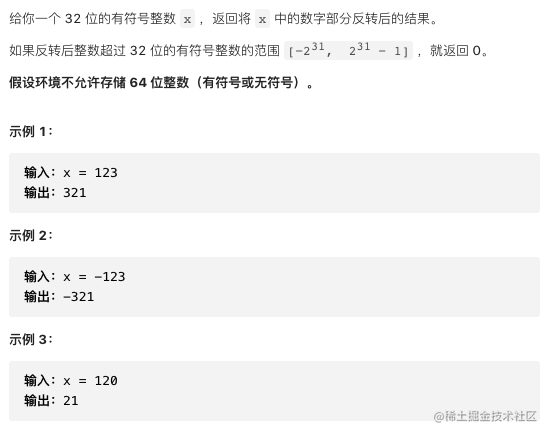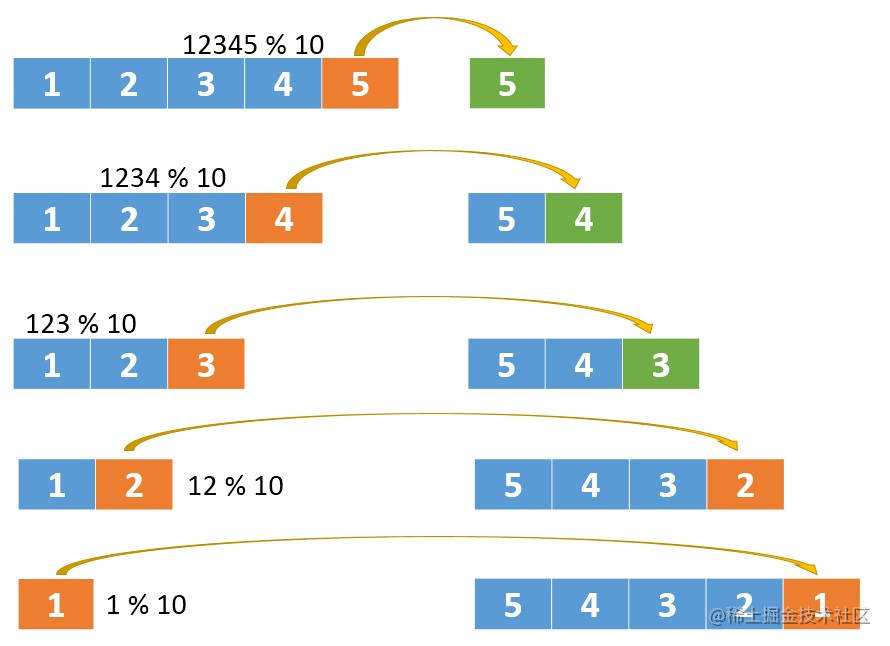``````var reverse = function(x) {
let ret = 0;
while(x){
ret = ret * 10 + x % 10;
if(ret > Math.pow(2, 31) - 1 || ret < Math.pow(-2, 31)) return 0;
x = (x / 10) | 0
}
return ret
};

## 141. 环形链表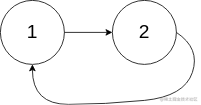``````输入： head = [3,2,0,-4], pos = 1

``````输入： head = [1,2], pos = 0

``````var hasCycle = function(head) {
while(traversingNode){
if(traversingNode.isVistitd) return true
traversingNode.isVistitd = true
traversingNode = traversingNode.next
}
return false;
};

## 160. 相交链表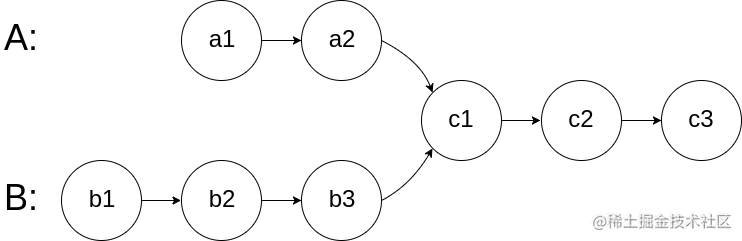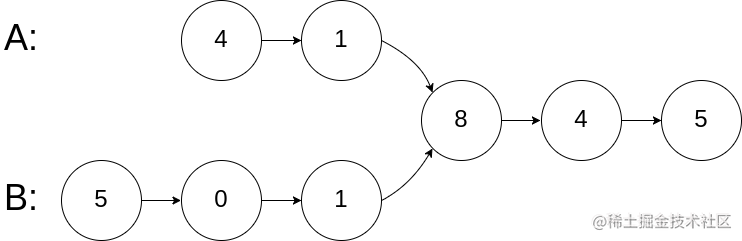``````输入：intersectVal = 8, listA = [4,1,8,4,5], listB = [5,0,1,8,4,5], skipA = 2, skipB = 3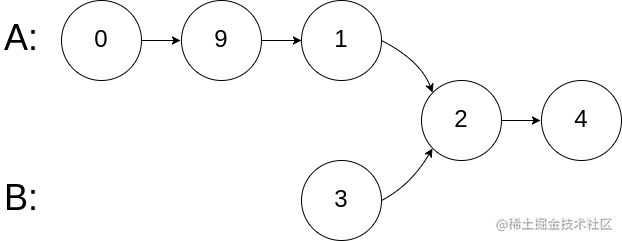``````输入：intersectVal = 2, listA = [0,9,1,2,4], listB = [3,2,4], skipA = 3, skipB = 1

## 202. 快乐数

「快乐数」定义为：

• 对于一个正整数，每一次将该数替换为它每个位置上的数字的平方和。
• 然后重复这个过程直到这个数变为 1，也可能是 无限循环 但始终变不到 1。
• 如果 可以变为  1，那么这个数就是快乐数。
• 如果 n 是快乐数就返回 true ；不是，则返回 false 。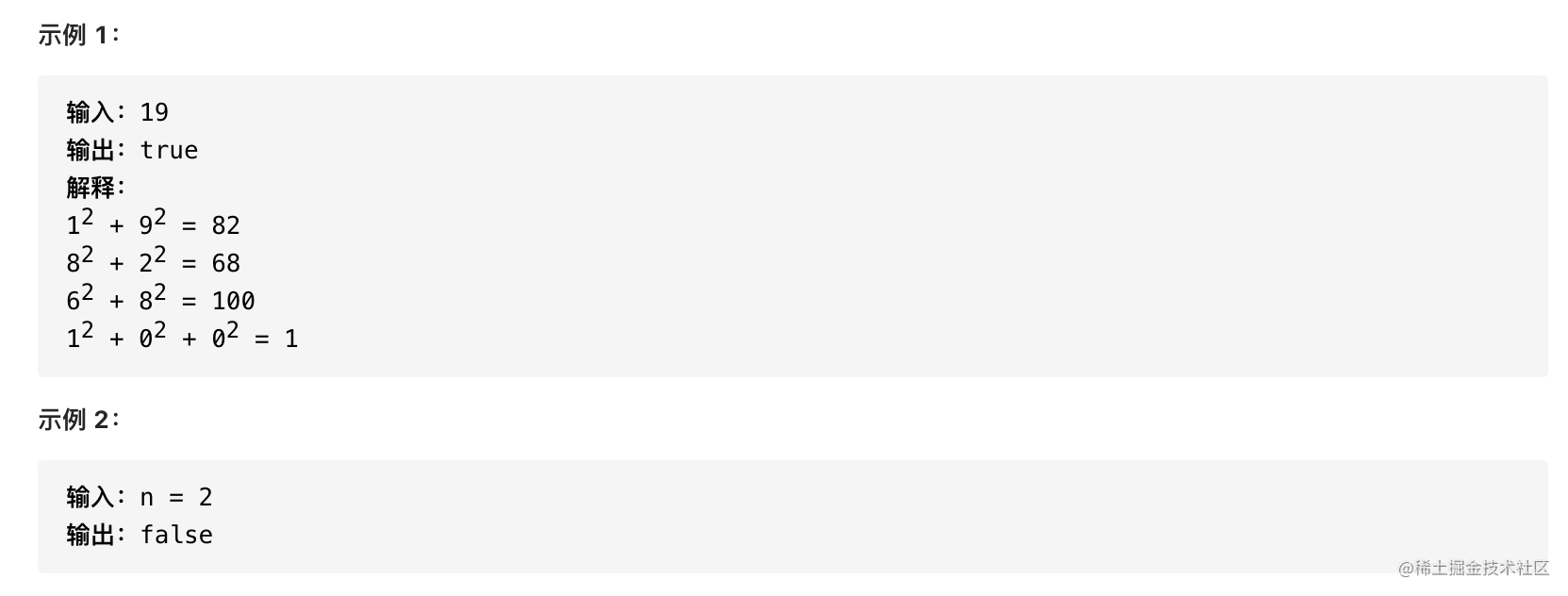1. 得到`1`
1. 无限循环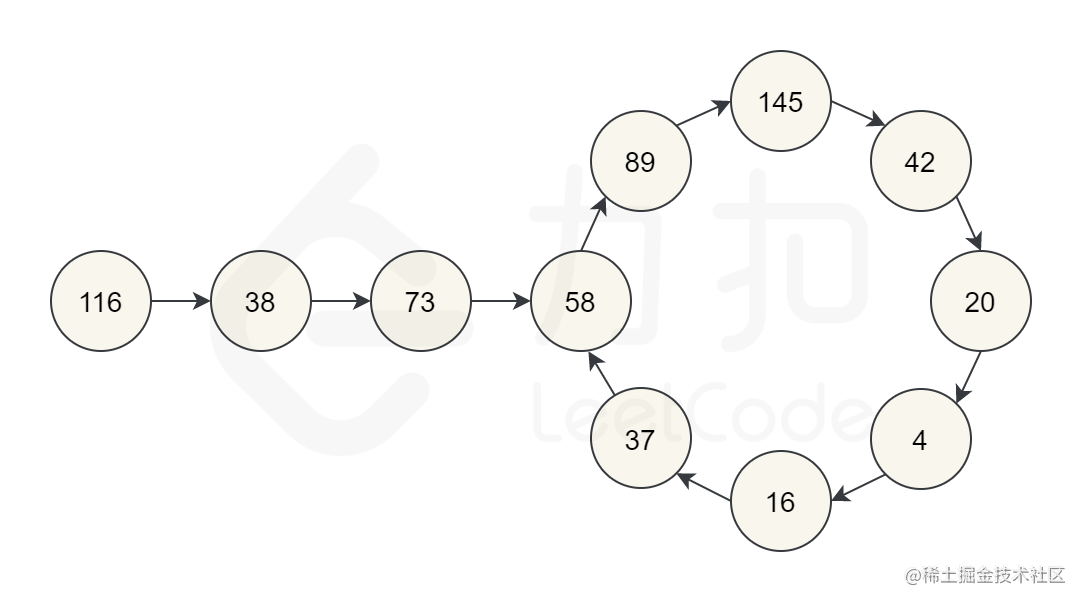• 可以看到如果你是13位，你的下一次快乐数算法会变为4位1053，
• 如果你是`9999`, `4`位，下一个快乐数是`324`

1981
299162
3999243
49999324
1399999999999991053

``````// 封装获取快乐数的方法
function getNext(n){
n = String(n);
let sum = 0;
for(let num of n){
sum = sum + Math.pow(+num, 2);
}
return sum;
}
var isHappy = function(n) {
// 哈希表来看是否循环
const map = {};
while( n !== 1 ){
map[n] = true;
n = getNext(n)
if(map[n]) return false
}
return true
};